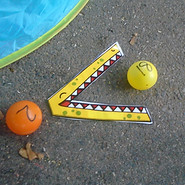## MathsFundamentally our aim is for everyone to enjoy maths. We challenge anyone’s notion that “I can’t do maths”. We can all enjoy games, activities and solving problems. Maths learning is engaging and inspiring at our school.

Our achievements can be seen in how we make progress in solving maths problems. We are curious, make connections and develop our resilience – aiming to get a little better at maths each day.

Our aim is to help children develop the ability to think mathematically. This lifelong skill is invaluable for us today and in an ever changing future.

###### Enjoy###### Achieve###### Aspire

Our principles of maths teaching and learning

Our curriculum is based on some key principles:

• All children can achieve.

• Many lessons keep the class working together so that all can access and master mathematics.

• Many lessons engage the whole class through a ‘low threshold’ so all can access. Further, the lessons are crafted to achieve a ‘high ceiling’ - Expectations should be high to challenge all appropriately.

• The focus is the development of factual fluency and deep mathematical understanding, shown through reasoning and problem solving.

• Children secure their understanding of abstract concepts through firstly using ‘concrete manipulatives’ and then pictorial representations.

• A longer time is spent on key topics, providing time to go deeper and embed learning.

• We use the Long Term Planning structure created by White Rose Maths https://whiterosemaths.com

• We learn by using the school’s learner tools: be curious, make connections, get involved, be reflective and be resilient.

(Many of these principles have been informed by our research into ‘The Mastery Approach’ to mathematics – more information can be found at www.ncetm.org.uk/resources/49450Online Learning Platforms

We are always looking for ways to help you support your child with improving their fluency of vital number facts and increase their progress in maths. We have subscribed to two on-line learning platforms called Sumdog and TTRockstars. Children play these apps and practice their maths skills.

Important age-related number fact expectations from the National Curriculum

Year 1

• count, read and write numbers to 100; count in multiples of twos, fives and tens

• represent and use number bonds and subtraction facts within 20

Year 2

• recognise the place value of each digit in a two-digit number (tens, ones)

• recall and use multiplication and division facts for the 2, 5 and 10 multiplication tables, including recognising odd and even numbers

Year 3

• recognise the place value of each digit in a three-digit number (hundreds, tens, ones)

• recall and use multiplication and division facts for the 3, 4 and 8 multiplication tables

Year 4

• recognise the place value of each digit in a four-digit number (thousands, hundreds, tens, and ones)

• recall multiplication and division facts for multiplication tables up to 12 × 12
(These are assessed by the national Year 4 Multiplication Tables Check)

Year 5

• read, write, order and compare numbers to at least 1 000 000 and determine the value of each digit

• multiply and divide whole numbers and decimals by 10, 100 and 1000

Year 6

• read, write, order and compare numbers up to 10 000 000 and determine the value of each digit

• multiply and divide numbers up to 4 digits by a two-digit whole number using the formal written method (see our progression in methods document)Our Calculation Progression

Please find below our school's progression in how we expect children to complete calculations for the four operations. We do not have an age-expectation for each stage. We strive for each child to find an efficient method for them at their current stage of learning.  Nevertheless, it should be noted that the National Curriculum expects children to use the formal written method in Year 6.For children

There are so many wonderful books available that explore maths concepts whilst telling a story or entertaining children. Here are some examples:

• 'Mathmagicians: How Maths Applies to Everything' by Jonny Ball

For parents/carers

• ‘Maths for Mums and Dads’ by Rob Eastaway